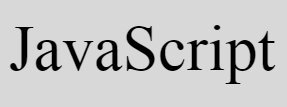# JavaScript Program to Check Vowel or Consonant

« Previous Program CodesCracker »

In this article, you will learn and get code in JavaScript to check whether a character entered by user is a vowel or consonant. Here are the list of JavaScript programs available in this article:

• Simple JavaScript Code with HTML to Check Vowel or Consonant
• Modified Version of above program to handle an invalid character
• Allow User to Enter the Character and then check for vowel or consonant

## Check Vowel or Consonant

This is a simplest JavaScript code to check for vowel. That is, this program checks the value stored in ch variable, whether it is a vowel or consonant.

```<!doctype html>
<html>
<body>
<script>
var ch='c';
if(ch=='a' || ch=='e' || ch=='i' || ch=='o' || ch=='u')
document.write("<b>"+ch+"</b>" + " is a Vowel");
else if(ch=='A' || ch=='E' || ch=='I' || ch=='O' || ch=='U')
document.write("<b>"+ch+"</b>" + " is a Vowel");
else
document.write("<b>"+ch+"</b>" + " is a Consonant");
</script>
</body>
</html>```

Save this code in a file with .html extension. Open the file in a web browser. Here is the output you will see:

Now change the value of ch with K. That is, initialize K to ch and re-open the file or refresh the web browser. Here is the output:

Note - The document.write() method writes into an HTML output.

### Program to Handle an Invalid Character

If user enters a number or any special character, then previous JavaScript program produces the output as a consonant, which is a wrong output.

So to handle these type of characters, here is the modified version of above JavaScript program to check vowel or not (consonant or any other character):

```<!doctype html>
<html>
<body>
<script>
var ch='9';
if((ch>='a' && ch<='z') || (ch>='A' && ch<='Z'))
{
if(ch=='a' || ch=='e' || ch=='i' || ch=='o' || ch=='u')
document.write("<b>"+ch+"</b>" + " is a Vowel");
else if(ch=='A' || ch=='E' || ch=='I' || ch=='O' || ch=='U')
document.write("<b>"+ch+"</b>" + " is a Vowel");
else
document.write("<b>"+ch+"</b>" + " is a Consonant");
}
else
document.write("<b>"+ch+"</b>" + " is neither Vowel nor Consonant");
</script>
</body>
</html>```

Here is the sample run of this program with user input, 9:

### Receive Character Input from User

This is the complete version of checking vowel or consonant using JavaScript program. As this program receives character input from user through HTML TextBox. Then checks and prints whether it is a vowel, consonant, or any other character as shown in the program given below:

```<!doctype html>
<html>
<script>
var ch;
function checkVowel()
{
ch = document.getElementById("char").value;
if(ch)
{
temp = document.getElementById("resPara");
temp.style.display = "block";
if((ch>='a' && ch<='z') || (ch>='A' && ch<='Z'))
{
if(ch=='a' || ch=='e' || ch=='i' || ch=='o' || ch=='u')
document.getElementById("res").innerHTML = "a Vowel";
else if(ch=='A' || ch=='E' || ch=='I' || ch=='O' || ch=='U')
document.getElementById("res").innerHTML = "a Vowel";
else
document.getElementById("res").innerHTML = "a Consonant";
}
else
document.getElementById("res").innerHTML = "neither Vowel nor Consonant";
}
}
</script>
<body>

<p>Enter the Character: <input id="char"><button onclick="checkVowel()">Check</button></p>
<p id="resPara" style="display:none;">It is <span id="res"></span></p>

</body>
</html>```

Here is its sample output:

Now supply any character say U as input and click on the Check button. Here is the output produced after performing these things:

The following code:

`style="display:none;"`

is a CSS code, used to hide an HTML element where it is present.

When user clicks on the button Check, a function checkVowel() gets called. The following JavaScript statement:

`ch = document.getElementById("char").value;`

states that, the value of an HTML element whose id is char gets initialized to ch. And the following JavaScript code/statement:

`document.getElementById("res").innerHTML = "a Vowel";`

states that, the value a Vowel gets written as an HTML element's output whose id is res.

#### Live Output of Above Program

Here is the live output produced by previous JavaScript program that is created to check whether the character entered (given) by user is a vowel, consonant, or any other.

Enter the Character:

JavaScript Online Test

« Previous Program CodesCracker »